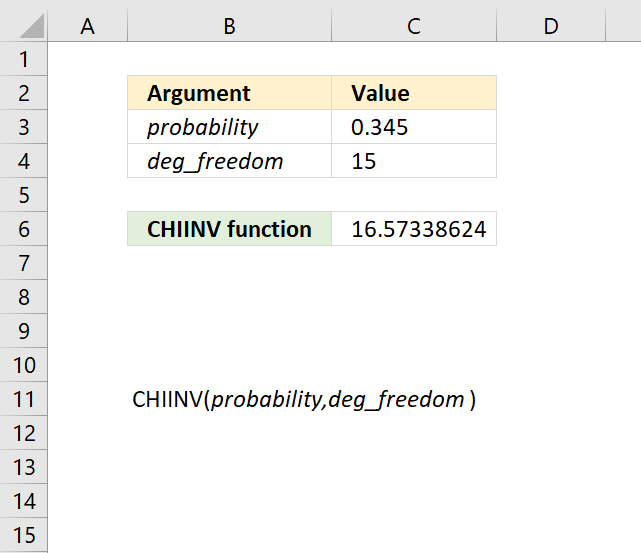Author: Oscar Cronquist Article last updated on September 27, 2018The CHIINV function calculates the inverse probability of the chi-squared distribution.

The CHIINV function is outdated and you will find it in the compatibility category, the function has been replaced by the CHISQ.INV function and the CHISQ.INV.RT function.

Formula in cell C6:

=CHIINV(C3,C4)

### Excel Function Syntax

CHIINV(probability,deg_freedom)

### Arguments

 probability Required. A numerical value representing the probability with the chi-squared distribution. deg_freedom Required. A numerical value representing the degrees of freedom.